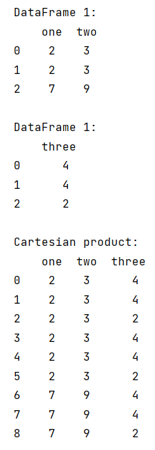# How to perform cartesian product in pandas?

Given two Pandas DataFrames, we have to perform cartesian product.
Submitted by Pranit Sharma, on June 26, 2022

Pandas is a special tool that allows us to perform complex manipulations of data effectively and efficiently. Inside pandas, we mainly deal with a dataset in the form of DataFrame. DataFrames are 2-dimensional data structures in pandas. DataFrames consists of rows, columns, and the data.

And since a DataFrame consists of rows and columns, it is easy to understand and analyze the data or we can say that pandas provide a slippery way to learn and to deliver a product in the form of an analyzed data set.

## Problem statement

When we have a data set in the form of a 2-dimensional DataFrame, we sometimes need to perform a cartesian product. Cartesian product is a special product where each row value of one array is multiplied by each column of another array, but in pandas, each element from the row will be added to a new DataFrame column-wise.

## Performing cartesian product in pandas

We can perform cartesian product in pandas by using the merge() method with a required parameter called 'how=cross'.

Note

To work with pandas, we need to import pandas package first, below is the syntax:

```import pandas as pd
```

Let us understand with the help of an example,

## Python code to perform cartesian product in pandas

```# Importing pandas package
import pandas as pd

# Creating a dictionary
d1 = {'one':[2,2,7],'two':[3,3,9]}
d2 = {'three':[4,4,2]}

# Creating two Dataframes
df1 = pd.DataFrame(d1)
df2 = pd.DataFrame(d2)

# Display DataFrames
print("DataFrame 1:\n",df1,"\n")
print("DataFrame 1:\n",df2,"\n")

# Cartesian product
result = df1.merge(df2, how='cross')

# Display result
print("Cartesian product:\n",result)
```

## Output